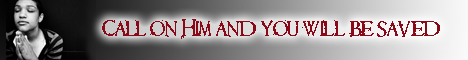Note:  Do not rely on this information. It is very old.

# Approximation

Approximation, a mathematical calculation that is not absolutely correct, but sufficiently near for certain practical purposes. Thus the circumference of a circle of unit diameter is approximately 22/7; the exact number cannot be expressed with a finite number of figures, and the importance of a useful approximation is obvious. Too high a degree of accuracy is needless in practical calculation, and involves waste of labour. Hence the practical utility of abridged methods of multiplication and division, of logarithmic and trigonometrical tables, which all involve approximations.